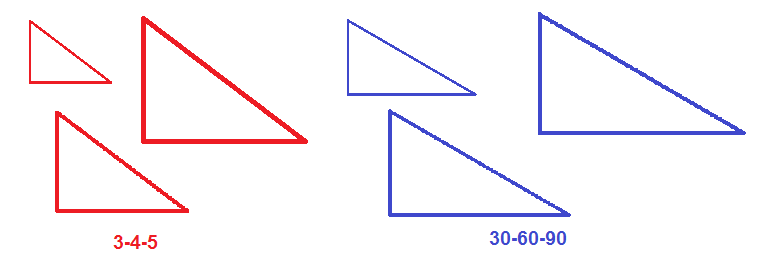### GRE Math MisconceptionsYou can attend the first session of any of our online or in-person GRE courses absolutely free. Crazy, right? Check out our upcoming courses here.

Math can be counterintuitive. There are a few GRE Math misconceptions that really seem like they should be true—but actually aren’t. Being prepared for them will keep you aware on test day.

Mistake: 1 is prime.

Fact: 1 isn’t prime. In fact, the smallest prime number is 2.

Why?: It seems like 1 should be prime, because you can’t divide it by any other integers. However, mathematicians have agreed to say that 1 isn’t a prime. This makes certain mathematical theorems much simpler and more intuitive. Even though you won’t use those theorems on the GRE (phew!), you have to deal with their consequences by remembering that 1 isn’t prime.

Mistake: 3-4-5 and 30-60-90 triangles are the same thing.

Fact: A right triangle can be 3-4-5 or 30-60-90, but not both.

Why?: Here’s a couple of 3-4-5 triangles next to a couple of 30-60-90 triangles. Even if the triangles get bigger or smaller, the triangles on the left all have different proportions from the triangles on the right. So, if the sides of a right triangle have the ratio 3-4-5, you know the angles aren’t 30-60-90, and vice versa.Mistake: If the ratio of teachers to students at a school is 1 to 4, then 1/4 of the people at the school are teachers.

Fact: In this scenario, only 1/5 of the people at the school are teachers!

Why?: A fraction always represents a part of a particular whole. In this case, the part is the number of teachers, and the whole is all of the people at the school. So, the denominator of the fraction has to be the sum of the teachers and the students, not just the students alone.

Try it out with numbers to confirm. If there are 10 teachers and  40 students, then 10 out of the 50 people at the school, or 1/5, are teachers.

Mistake: The average of the numbers from 1 to 10 is 5.

Fact: The average of the numbers from 1 to 10 is 5.5.

Why?: Intuition tells you that 5 is halfway from 1 to 10. However, to find the average of a bunch of consecutive numbers, you need to average the smallest and largest numbers together. The right answer will be the average of 1 and 10, which is (1+10)/2 = 11/2 = 5.5.

Confirm this by actually averaging the numbers from 1 to 10. Here’s the sum:

1+2+3+4+5+6+7+8+9+10 = 55

There are 10 terms, so the average is 55/10, which equals  5.5.

Mistake: If x is 25% greater than y, then y is 25% less than x.

Fact: If x is 25% greater than y, then y is only 20% less than x.

Why?: This is one of the most counterintuitive math facts out there, but the numbers back it up. Suppose that a coat costs 25% more than a sweater. If the sweater costs $100, the coat would cost 1.25($100), or $125. However, if a sweater costs 25% less than a coat, and the coat costs$125, the sweater only costs 0.75($125) =$93.75.

‘Percent more than’ and ‘percent less than’ aren’t interchangeable. Pay close attention to which term the problem actually uses. If it says ‘percent more’ or ‘percent greater,’ then use a decimal greater than 1, such as the 1.25 figure from the example above. If it says ‘percent less’ or ‘percent smaller,’ then use a decimal lower than 1, such as 0.75.

You can also prove this specific example using fractions. If x is 25% greater than y, then x is 5/4 of y. Use algebra rules to get y by itself:

x = 5/4 y

4x = 5y

4/5 x = y

y is fourth-fifths as large as x. Since the missing 1/5 is equivalent to 20%, y is only 20% smaller than x. 📝

See that “SUBSCRIBE” button in the top right corner? Click on it to receive all our GRE blog updates straight to your inbox!

Chelsey Cooleyis a Manhattan Prep instructor based in Seattle, Washington. Chelsey always followed her heart when it came to her education. Luckily, her heart led her straight to the perfect background for GMAT and GRE teaching: she has undergraduate degrees in mathematics and history, a master’s degree in linguistics, a 790 on the GMAT, and a perfect 170Q/170V on the GRE. Check out Chelsey’s upcoming GRE prep offerings here.

1.Elizabeth June 22, 2018 at 3:42 am

I now see that this, among similar concerns, may be addressed on the ETS website in the Math Conventions PDF. Thanks!

2.Elizabeth June 21, 2018 at 9:24 pm

Would a question on the GRE be similar to your example “Mistake: If the ratio of teachers to students at a school is 1 to 4, then 1/4 of the people at the school are teachers.” My concern is that we are not certain that all “people” in the school are either student or teacher. I am in the very beginning of my GRE studies so forgive me if this is an amateur question.